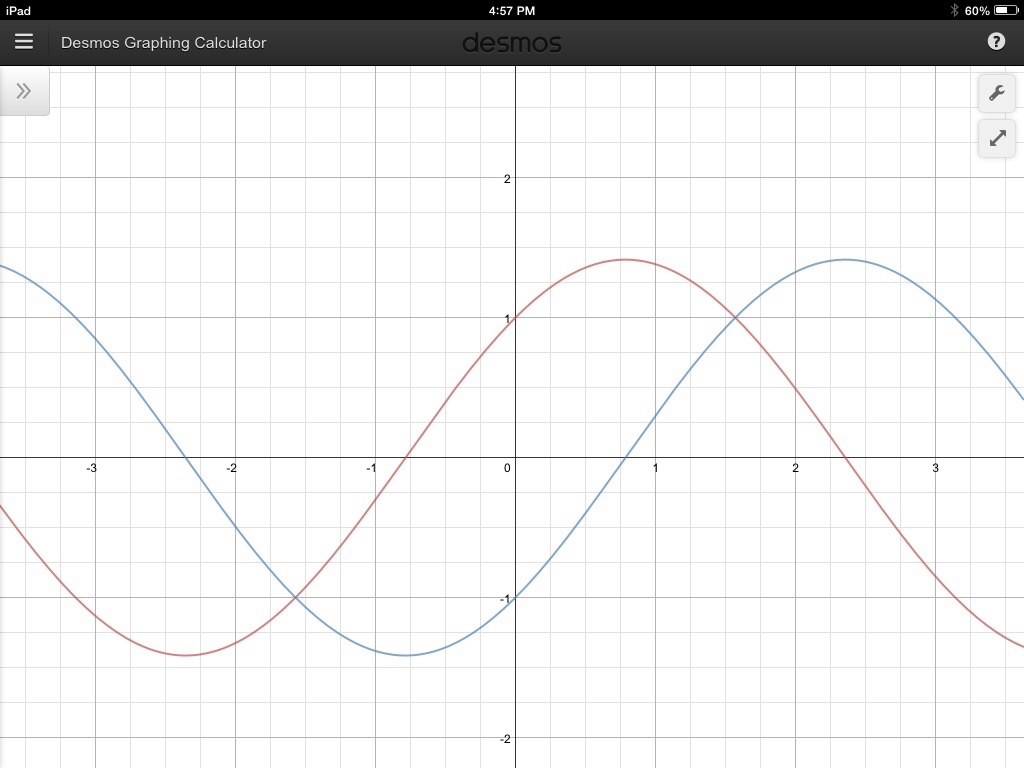# Looks like a bird...

Calculus Level 4These are the graphs of $\sin x +\cos x$ and $\sin x -\cos x$

Let the area of every region enclosed by the two curves be $A$

Find $A^2$

This is part of the Sinusoidal, Cosinusoidal series

×